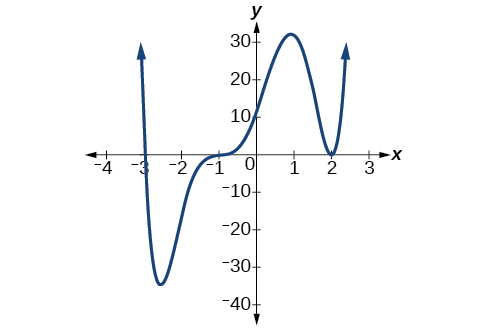# 5.3 Graphs of polynomial functions  (Page 2/13)

 Page 2 / 13

## Finding the x -intercepts of a polynomial function by factoring

Find the x -intercepts of $\text{\hspace{0.17em}}f\left(x\right)={x}^{6}-3{x}^{4}+2{x}^{2}.$

We can attempt to factor this polynomial to find solutions for $\text{\hspace{0.17em}}f\left(x\right)=0.$

$\begin{array}{ccccccccccc}& & & & \hfill \left({x}^{2}-1\right)& =& 0\hfill & & \hfill \left({x}^{2}-2\right)& =& 0\hfill \\ \hfill {x}^{2}& =& 0\hfill & \phantom{\rule{2em}{0ex}}\text{or}& \hfill {x}^{2}& =& 1\hfill & \phantom{\rule{2em}{0ex}}\text{or}& \hfill {x}^{2}& =& 2\hfill \\ \hfill x& =& 0\hfill & & \hfill x& =& ±1\hfill & & \hfill x& =& ±\sqrt{2}\hfill \end{array}$

This gives us five x -intercepts: $\text{\hspace{0.17em}}\left(0,0\right),\left(1,0\right),\left(-1,0\right),\left(\sqrt{2},0\right),\text{\hspace{0.17em}}$ and $\text{\hspace{0.17em}}\left(-\sqrt{2},0\right).\text{\hspace{0.17em}}$ See [link] . We can see that this is an even function because it is symmetric about the y -axis.

## Finding the x -intercepts of a polynomial function by factoring

Find the x -intercepts of $\text{\hspace{0.17em}}f\left(x\right)={x}^{3}-5{x}^{2}-x+5.$

Find solutions for $\text{\hspace{0.17em}}f\left(x\right)=0\text{\hspace{0.17em}}$ by factoring.

$\begin{array}{ccccccccccc}\hfill x+1& =& 0\hfill & \phantom{\rule{2em}{0ex}}\text{or}\phantom{\rule{2em}{0ex}}& \hfill x-1& =& 0\hfill & \phantom{\rule{2em}{0ex}}\text{or}\phantom{\rule{2em}{0ex}}& \hfill x-5& =& 0\hfill \\ \hfill x& =& -1\hfill & & \hfill x& =& 1\hfill & & \hfill x& =& 5\hfill \end{array}$

There are three x -intercepts: $\text{\hspace{0.17em}}\left(-1,0\right),\left(1,0\right),\text{\hspace{0.17em}}$ and $\text{\hspace{0.17em}}\left(5,0\right).\text{\hspace{0.17em}}$ See [link] .

## Finding the y - and x -intercepts of a polynomial in factored form

Find the y - and x -intercepts of $\text{\hspace{0.17em}}g\left(x\right)={\left(x-2\right)}^{2}\left(2x+3\right).$

The y -intercept can be found by evaluating $\text{\hspace{0.17em}}g\left(0\right).$

$\begin{array}{ccc}\hfill g\left(0\right)& =& {\left(0-2\right)}^{2}\left(2\left(0\right)+3\right)\hfill \\ & =& 12\hfill \end{array}$

So the y -intercept is $\text{\hspace{0.17em}}\left(0,12\right).$

The x -intercepts can be found by solving $\text{\hspace{0.17em}}g\left(x\right)=0.$

${\left(x-2\right)}^{2}\left(2x+3\right)=0$
$\begin{array}{ccccccc}\hfill {\left(x-2\right)}^{2}& =& 0\hfill & & \hfill \left(2x+3\right)& =& 0\hfill \\ \hfill x-2& =& 0\hfill & \phantom{\rule{2em}{0ex}}\text{or}\phantom{\rule{2em}{0ex}}& \hfill x& =& -\frac{3}{2}\hfill \\ \hfill x& =& 2\hfill & & & & \end{array}$

So the x -intercepts are $\text{\hspace{0.17em}}\left(2,0\right)\text{\hspace{0.17em}}$ and $\text{\hspace{0.17em}}\left(-\frac{3}{2},0\right).$

## Finding the x -intercepts of a polynomial function using a graph

Find the x -intercepts of $\text{\hspace{0.17em}}h\left(x\right)={x}^{3}+4{x}^{2}+x-6.$

This polynomial is not in factored form, has no common factors, and does not appear to be factorable using techniques previously discussed. Fortunately, we can use technology to find the intercepts. Keep in mind that some values make graphing difficult by hand. In these cases, we can take advantage of graphing utilities.

Looking at the graph of this function, as shown in [link] , it appears that there are x -intercepts at $\text{\hspace{0.17em}}x=-3,-2,\text{\hspace{0.17em}}$ and $\text{\hspace{0.17em}}1.$

We can check whether these are correct by substituting these values for $\text{\hspace{0.17em}}x\text{\hspace{0.17em}}$ and verifying that

$h\left(-3\right)=h\left(-2\right)=h\left(1\right)=0$

Since $\text{\hspace{0.17em}}h\left(x\right)={x}^{3}+4{x}^{2}+x-6,\text{\hspace{0.17em}}$ we have:

$\begin{array}{ccc}\hfill h\left(-3\right)& =& {\left(-3\right)}^{3}+4{\left(-3\right)}^{2}+\left(-3\right)-6=-27+36-3-6=0\hfill \\ \hfill h\left(-2\right)& =& {\left(-2\right)}^{3}+4{\left(-2\right)}^{2}+\left(-2\right)-6=-8+16-2-6=0\hfill \\ \hfill h\left(1\right)& =& {\left(1\right)}^{3}+4{\left(1\right)}^{2}+\left(1\right)-6=1+4+1-6=0\hfill \end{array}$

Each x -intercept corresponds to a zero of the polynomial function and each zero yields a factor, so we can now write the polynomial in factored form.

$\begin{array}{ccc}\hfill h\left(x\right)& =& {x}^{3}+4{x}^{2}+x-6\hfill \\ & =& \left(x+3\right)\left(x+2\right)\left(x-1\right)\hfill \end{array}$

Find the y - and x -intercepts of the function $\text{\hspace{0.17em}}f\left(x\right)={x}^{4}-19{x}^{2}+30x.$

y -intercept $\text{\hspace{0.17em}}\left(0,0\right);\text{\hspace{0.17em}}$ x -intercepts $\text{\hspace{0.17em}}\left(0,0\right),\left(–5,0\right),\left(2,0\right),\text{\hspace{0.17em}}$ and $\text{\hspace{0.17em}}\left(3,0\right)$

## Identifying zeros and their multiplicities

Graphs behave differently at various x -intercepts. Sometimes, the graph will cross over the horizontal axis at an intercept. Other times, the graph will touch the horizontal axis and "bounce" off.

Suppose, for example, we graph the function shown.

$f\left(x\right)=\left(x+3\right){\left(x-2\right)}^{2}{\left(x+1\right)}^{3}$

Notice in [link] that the behavior of the function at each of the x -intercepts is different.Identifying the behavior of the graph at an x -intercept by examining the multiplicity of the zero.

The x -intercept $\text{\hspace{0.17em}}x=-3\text{\hspace{0.17em}}$ is the solution of equation $\text{\hspace{0.17em}}\left(x+3\right)=0.\text{\hspace{0.17em}}$ The graph passes directly through the x -intercept at $\text{\hspace{0.17em}}x=-3.\text{\hspace{0.17em}}$ The factor is linear (has a degree of 1), so the behavior near the intercept is like that of a line—it passes directly through the intercept. We call this a single zero because the zero corresponds to a single factor of the function.

how do I set up the problem?
what is a solution set?
Harshika
hello, I am happy to help!
Abdullahi
find the value of 2x=32
divide by 2 on each side of the equal sign to solve for x
corri
X=16
Michael
Want to review on complex number 1.What are complex number 2.How to solve complex number problems.
Beyan
use the y -intercept and slope to sketch the graph of the equation y=6x
how do we prove the quadratic formular
hello, if you have a question about Algebra 2. I may be able to help. I am an Algebra 2 Teacher
thank you help me with how to prove the quadratic equation
Seidu
may God blessed u for that. Please I want u to help me in sets.
Opoku
what is math number
4
Trista
x-2y+3z=-3 2x-y+z=7 -x+3y-z=6
Need help solving this problem (2/7)^-2
x+2y-z=7
Sidiki
what is the coefficient of -4×
-1
Shedrak
the operation * is x * y =x + y/ 1+(x × y) show if the operation is commutative if x × y is not equal to -1
An investment account was opened with an initial deposit of \$9,600 and earns 7.4% interest, compounded continuously. How much will the account be worth after 15 years?
lim x to infinity e^1-e^-1/log(1+x)
given eccentricity and a point find the equiationByByBy Tony PizurBy OpenStaxBy OpenStaxByBy Darlene PaliswatBy OpenStaxBy Rylee MinllicBy OpenStaxBy Jazzycazz JacksonBy OpenStax